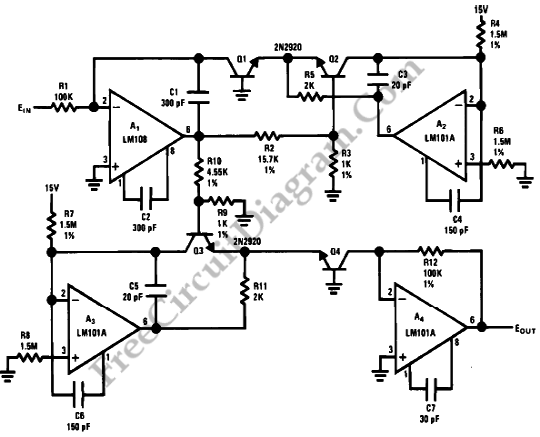# Power Function CircuitWe can generate easily many non-linear functions such as X^1/2, X^2, X^3, 1/X, XY and X/Y using logs. Division becomes subtraction, multiplication becomes addition and powers become gain coefficients of log terms. The circuit diagram below shows a circuit whose output is the cube of the input. Actually, by changing the values of R9 and R10 in accordance with the expression Eout= Ein^[16.7R9/(R9+R10)] any power function is available from this circuit.But, we have to take a note that when log and anti-log circuits are used to perform an operation with a linear output, no temperature compensating resistors at all are needed since they cancel each other the non-linearity error. Gain changes with temperature cancel if the log and anti-log transistors are at the same temperature.To minimize thermal gradients, it’s a good idea to use a heat sink which couples the two transistors. A 1’C temperature difference between the log and anti-log transistors results in a 0.3% error. Also a 1’C temperature difference between the log transistors and the compensating resistor results in a 0.3% error in the log converters. [Source: National Semiconductor Application Note]